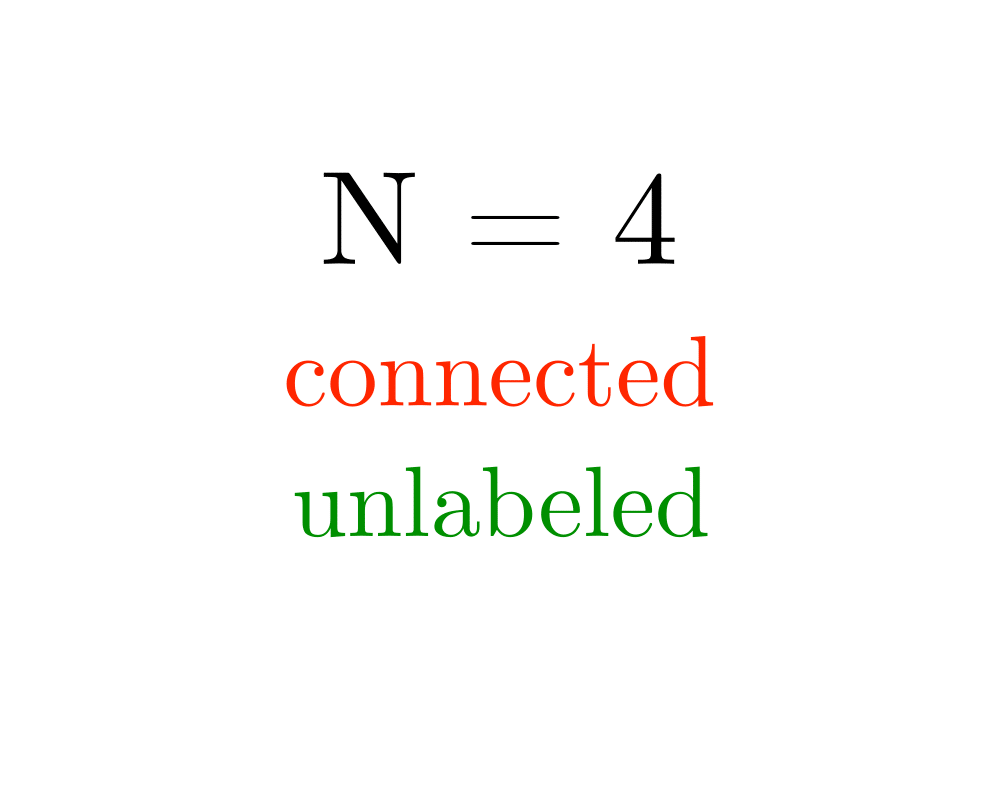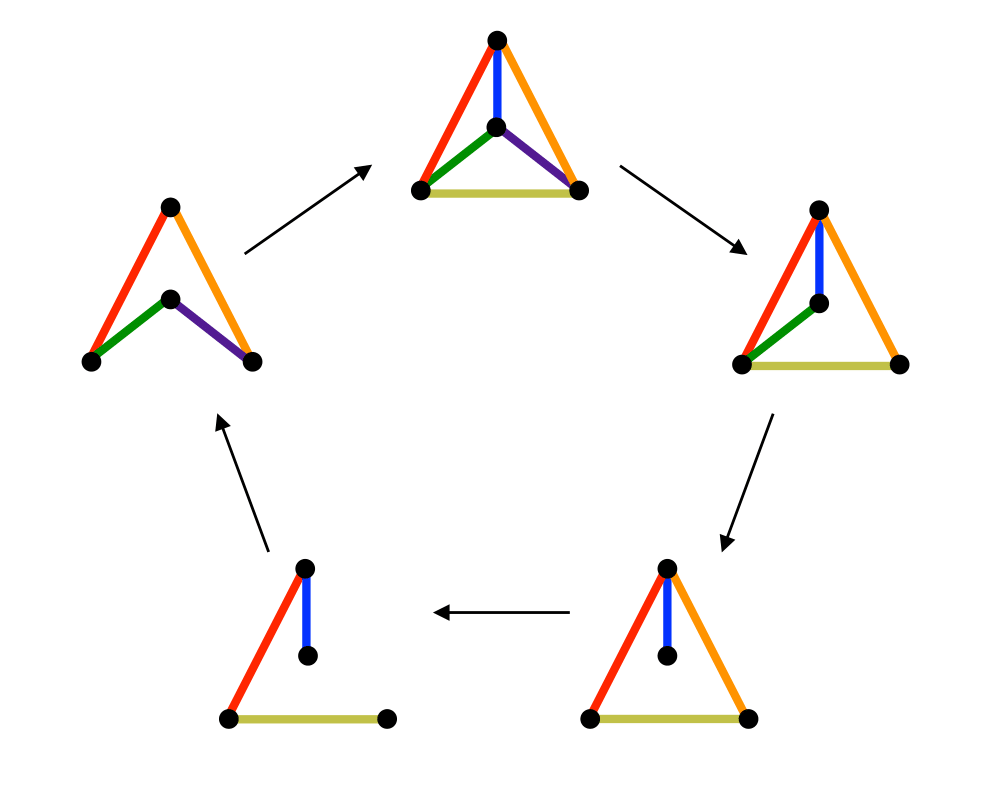# Generating GraphsInputOutput

Input Description: Parameters describing the desired graph, such as the number of vertices $$n$$, the number of edges $$m$$, or the edge probability $$p$$.
Problem: Generate (1) all, or (2) a random, or (3) the next graph satisfying the parameters.

Excerpt from The Algorithm Design Manual: Graph generation typically arises in constructing test data for programs. Perhaps you have two different programs that solve the same problem, and you want to see which one is faster or make sure that they always give the same answer. Another application is experimental graph theory, verifying whether a particular property is true for all graphs or how often it is true. It is much easier to conjecture the four-color theorem once you have demonstrated 4-colorings for all planar graphs on 15 vertices.

A different application of graph generation arises in network design. Suppose you need to design a network linking ten machines using as few cables as possible, such that the network can survive up to two vertex failures. One approach is to test all the networks with a given number of edges until you find one that will work. For larger graphs, more heuristic approaches, like simulated annealing, will likely be necessary.

Go To Main Page